# The Rectified 24-cell

The rectified 24-cell is a uniform polychoron bounded by 48 cells (24 cuboctahedra, 24 cubes), 240 polygons (96 triangles, 144 squares), 288 edges, 96 vertices. It coincides with the cantellated 16-cell, due to the coincidence of the 24-cell with the rectified 16-cell.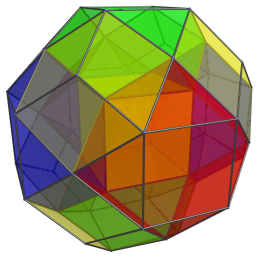The rectified 24-cell, as its name implies, is constructed by rectifying the 24-cell: truncating it to the midpoints of its edges. This operation reduces its octahedral cells into cuboctahedra, and introduces 24 cubical cells.

## Structure

We shall explore the structure of the rectified 24-cell by looking at its cube-first parallel projection into 3D.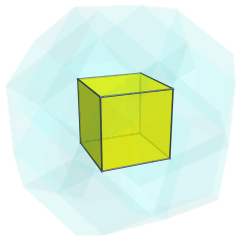The above image shows the cubical cell nearest to the 4D viewpoint. For clarity, we render the rest of the cells in a transparent color and omit edges and vertices not on this cube.

This cube is surrounded by 6 cuboctahedra, as shown next as 3 opposing pairs: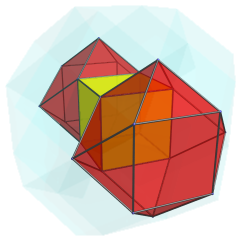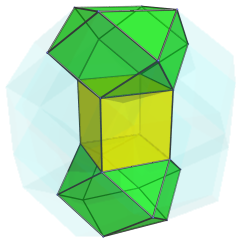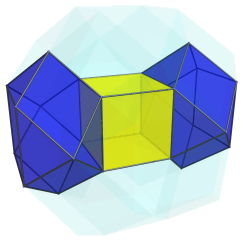These cuboctahedra appear to be slightly flattened, because they lie at a 45° angle to the 4D viewpoint. In 4D, they are perfectly uniform. The next image shows all of them together: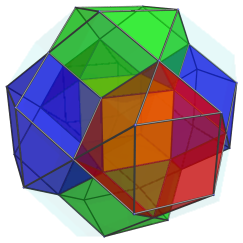In addition to these cuboctahedra, there are 8 cubes that touch the original cube's vertices. These are shown below: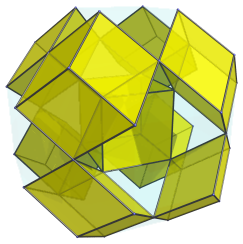As with the cuboctahedra, these cubes are seen at an angle, and thus appear foreshortened. They are perfectly regular in 4D. The following image shows them and the cuboctahedra together: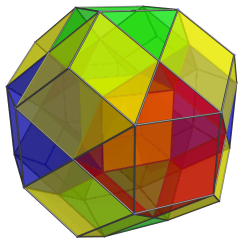These 9 cubes (including the first one) and 6 cuboctahedra lie on the “northern hemisphere” of the rectified 24-cell. Past this point, there are a number of cells on its “equator”: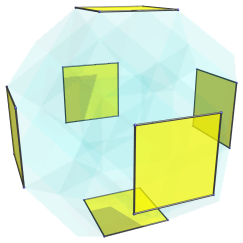For the sake of clarity, we omit the cells previously seen. These 6 squares are actually 6 cubes; they have been foreshortened into squares because they are seen at a 90° angle.

There are 12 cuboctahedra on the equator; these fill up the remaining gaps on the equator: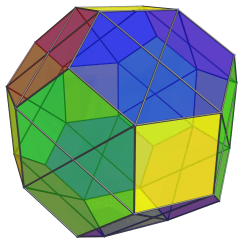Just like the cubes, these cuboctahedra are seen at a 90° angle, so they have been foreshortened into hexagons. In reality, they are perfectly uniform cuboctahedra. As can be clearly seen here, the projection envelope of the rectified 24-cell under the current cube-centered projection is a truncated rhombic dodecahedron.

After these equatorial cells, we have the cells in the “southern hemisphere” of the rectified 24-cell. These cells exactly mirror the arrangement as the northern hemisphere cells. Thus, in the northern hemisphere we have 1+8=9 cubes, on the equator we have 6 cubes, and in the southern hemisphere we have another 9 cubes, for a total of 24 cubes. There are 6 cuboctahedra in the northern hemisphere, 12 on the equator, and another 6 in the southern hemisphere, also totalling 24 cuboctahedra. In total, there are 48 cells.

## The Cantellated 16-cell

Due to the coincidence of the 24-cell as the rectified 16-cell, the rectified 24-cell is the same as the cantellated 16-cell. The symmetry of the cantellated 16-cell can be clearly seen when we examine the projections of the rectified 24-cell centered on a cuboctahedron: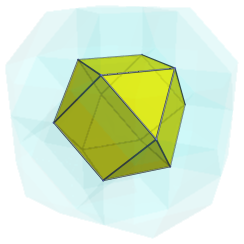This is the closest cuboctahedron to the 4D viewpoint. Its 6 square faces are joined to 6 cubes: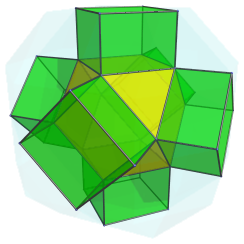The triangular faces of the nearest cuboctahedron are joined to another 8 cuboctahedra, shown below in two sets of four: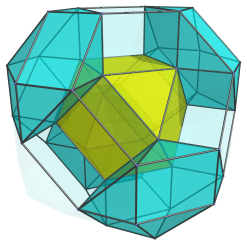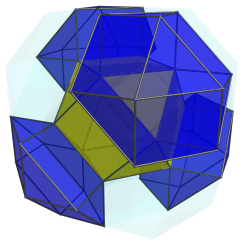Here are all 8 cuboctahedra together: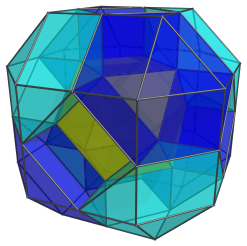And here are the 6 cubes added into the mix: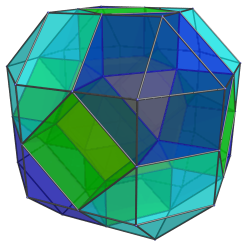These are all the cells that lie on the near side of the rectified 24-cell from this 4D viewpoint. On the limb of the rectified 24-cell are 6 more cuboctahedra, as shown below: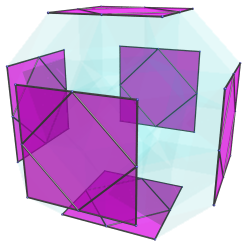For clarity, we omit the cells seen before. These cuboctahedra have been foreshortened into squares, because they are seen at a 90° angle. Joining these cuboctahedra are 12 cubes: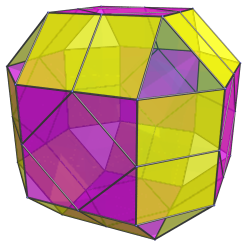These cubes are perfectly regular in 4D, but they appear flattened into rectangles because they are being seen from a 90° angle.

The remaining triangular gaps are not the images of any cells; they are the triangular face where the 8 cuboctahedra from the near side touch their corresponding counterparts on the far side of the rectified 24-cell. The structure of cells on the far side exactly mirrors the near side.

In total, there are 1+8=9 cuboctahedra on the near side, 6 cuboctahedra on the limb, and another 9 cuboctahedra on the far side, giving a total of 24 cuboctahedra. There are 6 cubes on the near side, 12 on the limb, and another 6 cubes on the far side; also totalling 24 cubes.

## Coordinates

The coordinates of the rectified 24-cell, which is the same as the cantellated 16-cell, are all permutations of coordinate and changes of sign of:

• (0, √2, √2, 2√2)

These coordinates give a rectified 24-cell centered on the origin with edge length 2.

Last updated 17 Jun 2019.